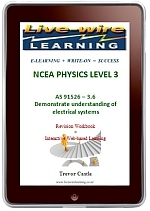### Physics AS 3.6 Electrical SystemsThe contents include:
Series and Parallel Circuits, Internal Resistance, Kirchhoff’s Circuit Laws, The Capacitor and Electric Field, Capacitance Construction, Capacitors in Series and Parallel, Capacitor Charge and Discharge through a Resistor, Electromagnetic Induction, The Inductor and Magnetic Field, Energy Stored in an Inductor, The Transformer, Resistor AC Circuit, Capacitor AC Circuit, Inductance AC Circuit, Resistor Capacitor and Resistor Inductance Series Circuit, Resistor Capacitor Inductance Series Circuit.

Price: \$17.95
Quantity: